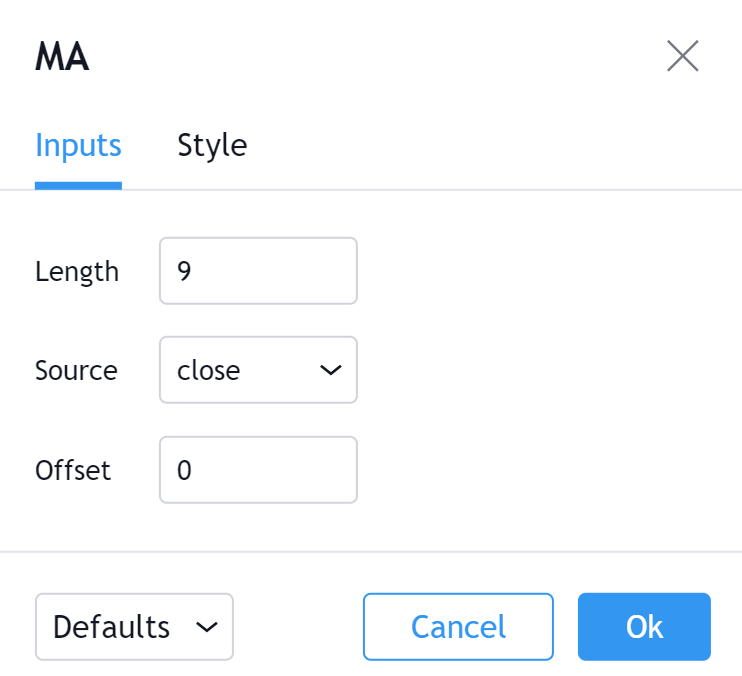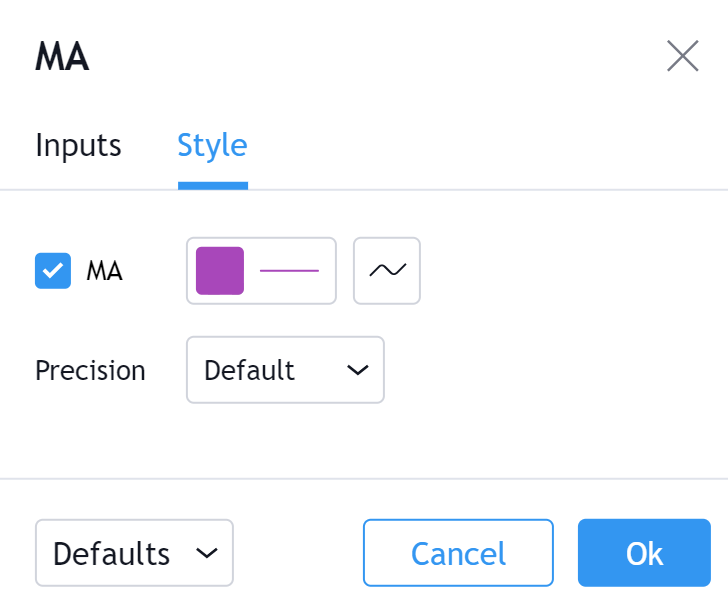# 移动平均线

3 周期 SMA 的示例

`周期值总和 / 周期数使用的收盘价：5, 6, 7, 8, 93周期SMA的第一天：(5 + 6 + 7) / 3 = 63周期SMA的第二天：(6 + 7 + 8) / 3 = 73周期SMA的第三天：(7 + 8 + 9) / 3 = 8`

`周期值总和 / 周期数使用的收盘价：5, 6, 7, 8, 9   周期        权重            价格          加权平均    1           1        X      5              5    2           2        X      6              12    3           3        X      7              21    4           4        X      8              32    5           5        X      9              45总计    =       15     X      35      =      525WMA = (加权平均总和) / (权重总和)WMA   115 / 15 = 7.6667`

1.计算SMA

`(周期值 / 周期数)`

2.计算乘数

`(2 / (周期数 + 1) 因此 (2 / (5+1) = 33.333%`

3.计算EMA

`EMA = {收盘价 - EMA(前一天)} x 乘数 + EMA(前一天)`

`双重 EMA = 2*EMA – EMA (EMA)`

`san'c EMA = (3*EMA – 3*EMA(EMA)) + EMA(EMA(EMA))`

• 明显上升的长期移动平均线是对看涨趋势的确认。
• 明显下降的长期移动平均线是对看跌趋势的确认。

##### 交叉MA